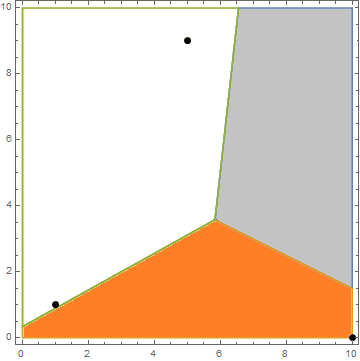시간 제한 메모리 제한 제출 정답 맞은 사람 정답 비율
15 초 512 MB 0 0 0 0.000%

## 문제

Alexander the Great is dead, and there is no legitimate heir. The empire is going to be divided into pieces between his generals. Nobody wants to start a bloody war, as they were friends just a while ago, so the generals are trying to invent some fair ways to draw the borders.

The empire could be represented as a rectangle on a plane with sides parallel to the coordinate axes. There are $N$ generals situated in different points inside this rectangle. Their first idea was to give everybody an area that is closer to him than to any other general. However, those of them who are now somewhere in the Middle East desert sharply rejected this way of division.

Next, the generals came up with an idea to ask a famous scientist how to distribute the land. They chose Euclid, as he was an outstanding mathematician of the ancient times. His first suggestion was to give everybody an area that is farther from him than from any other general. Help them to check this idea, calculate for each general the area of the land he will receive according to this distribution rule. Actually, generals are not interested in the precise values of the areas itself, but what is the percentage each general is going to receive.

## 입력

The first line of the input describes an empire by two pairs of integers $L$, $D$, and $R$, $U$, denoting bottom left and upper right corners of the rectangle respectively ($0 \leq |L|, |R|, |U|, |D| \leq 10^6$, $L < R$, $D < U$).

The second line contains a single integer $N$, the number of generals who want to cut a piece from the great legacy ($1 \leq N \leq 100\,000$).

The next $N$ lines describe the locations of generals. Each of these lines contains $x_i$ and $y_i$ --- coordinates of the point on a plane where $i$-th general is located ($L \leq x_i \leq R$, $D \leq y_i \leq U$). All the points are distinct.

## 출력

Print $N$ lines, $i$-th of them containing a single real value, denoting the share of $i$-th general, which is defined as the area of the part that $i$-th general will own according to the distribution suggested by Euclid, divided by the total area of the great empire. Your answer will be considered correct if its absolute error does not exceed $10^{-6}$.

Formally, if your answer for some general is $A$ and the jury's answer is $B$, the checker will accept your answer if $|A-B| \leq 10^{-6}$.

## 예제 입력 1

0 0 10 10
3
1 1
5 9
10 0


## 예제 출력 1

0.2883073300
0.2195372459
0.4927790674


## 힌트The picture corresponds to the example case.